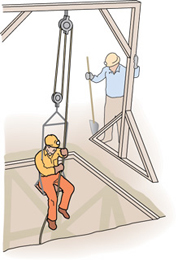# Problem: A man with mass exttip{m}{m} sits on a platform suspended from a movable pulley, as shown in the figure , and raises himself at constant speed by a rope passing over a fixed pulley. The platform and the pulleys have negligible mass. Assume that there are no friction losses.Find the force he must exert.Find the increase in the energy of the system when he raises himself a distance exttip{x}{x}. (Answer by calculating the increase in potential energy.)Find the increase in the energy of the system when he raises himself exttip{x}{x}. (Answer by computing the product of the force on the rope and the length of the rope passing through his hands.)

⚠️Our tutors found the solution shown to be helpful for the problem you're searching for. We don't have the exact solution yet.

###### Problem Details

A man with mass sits on a platform suspended from a movable pulley, as shown in the figure, and raises himself at constant speed by a rope passing over a fixed pulley. The platform and the pulleys have negligible mass. Assume that there are no friction losses.

Find the force he must exert.

Find the increase in the energy of the system when he raises himself a distance . (Answer by calculating the increase in potential energy.)

Find the increase in the energy of the system when he raises himself . (Answer by computing the product of the force on the rope and the length of the rope passing through his hands.)